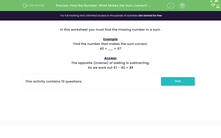# Understand How to Use Inverse Operation to Find a Missing Number

In this worksheet, students will find the missing number in a calculation using subtraction.Key stage:  KS 2

Curriculum topic:   Number: Addition and Subtraction

Curriculum subtopic:   Use Correct Methods to Problem Solve

Difficulty level:#### Worksheet Overview

In this activity, we will be finding the missing number in a calculation.

Example

Find the number that makes the calculation correct.

40 + __ = 67

The opposite (inverse) of adding is subtracting.

So we work out 67 - 40 = 27

Let's practise this skill in the following questions.### What is EdPlace?

We're your National Curriculum aligned online education content provider helping each child succeed in English, maths and science from year 1 to GCSE. With an EdPlace account you’ll be able to track and measure progress, helping each child achieve their best. We build confidence and attainment by personalising each child’s learning at a level that suits them.

Get started